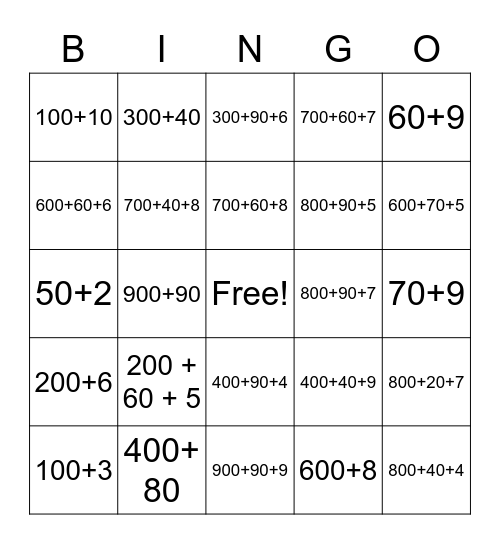# Break Apart BingoThis bingo card has a free space and 24 words: 200 + 60 + 5, 400+ 80, 700+60+8, 100+3, 60+9, 100+10, 600+60+6, 300+40, 800+90+7, 400+90+4, 800+90+5, 50+2, 400+40+9, 800+20+7, 700+40+8, 600+8, 70+9, 300+90+6, 600+70+5, 900+90, 800+40+4, 200+6, 700+60+7 and 900+90+9.

## Play Online